# 白话零知识证明（二）

2019/01/09 16:27

hi 欢迎来到小秘课堂第三期，今天我们来讲讲白话零知识证明（二）的那些事儿，欢迎主讲人马宇峰

讲师：马宇峰

# 举例说明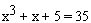（提示：解是 3，解用来构造 witness）。这个例子既可以让你理解 zk-SNARK 背后的技术原理，又不会产生出很大的 QAP.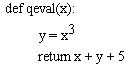1：x = y  ( y 可以是变量或者数字)

2：x = y op z  ( op 是二元运算符，可以是 (+, - , *, /) 运算，y，z 可以是变量，数字或者子表达式 )

“拍平”后的结果是：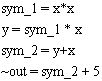R1CS 是一个由三向量组 (a,b,c) 组成的序列，R1CS 有个解向量 s，s 必须满足，符号表示向量的内积运算。这里的解向量 s 就是 witness。举个例子：

a = (5,0,0,0,0,1)，

b = (1,0,0,0,0,0)，

c = (0,0,1,0,0,0)，

s = (1,3,35,9,27,30)，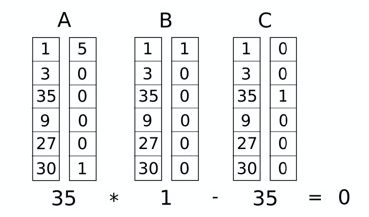'~one',' x', '~out', 'sym_1', 'y', 'sym_2' 解向量是以这个顺序对这些变量所赋的值。

'~one', ' x', '~out', 'sym_1', 'y', 'sym_2'

a = [0,  1,  0,  0,   0,  0]

b = [0,  1,  0,  0,   0,  0]

c = [0,  0,  0,  1,   0,  0]

，同样，如果 s 的第二个标量是 7，第四个标量是 49，也会通过检查，第一次检查仅仅是为了验证第一个门的输入和输出的一致性。

'~one', ' x', '~out', 'sym_1', 'y', 'sym_2'

a = [0,  0,  0,  1,   0,  0]

b = [0,  1,  0,  0,   0,  0]

c = [0,  0,  0,  0,   1,  0]

'~one', ' x', '~out', 'sym_1', 'y', 'sym_2'

a = [0,  1,  0,  0,   1,  0]

b = [1,  0,  0,  0,   0,  0]

c = [0,  0,  0,  0,   0,  1]

'~one', ' x', '~out', 'sym_1', 'y', 'sym_2'

a = [5,  0,  0,  0,   0,  1]

b = [1,  0,  0,  0,   0,  0]

c = [0,  0,  1,  0,   0,  0]

~out = sym_2 + 5.

witness（即解向量）是 [1,3,35,9,27,30]。(因为我们知道 x=3,将它带入“拍平”后的声明语句就可得到其他变量的解)。

A

[0, 1, 0, 0, 0, 0]

[0, 0, 0, 1, 0, 0]

[0, 1, 0, 0, 1, 0]

[5, 0, 0, 0, 0, 1]

B

[0, 1, 0, 0, 0, 0]

[0, 1, 0, 0, 0, 0]

[1, 0, 0, 0, 0, 0]

[1, 0, 0, 0, 0, 0]

C

[0, 0, 0, 1, 0, 0]

[0, 0, 0, 0, 1, 0]

[0, 0, 0, 0, 0, 1]

[0, 0, 1, 0, 0, 0]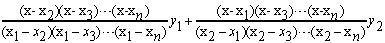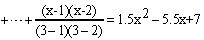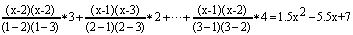A 多项式组

[-5.0, 9.166, -5.0, 0.833]

[8.0, -11.333, 5.0, -0.666]

[0.0, 0.0, 0.0, 0.0]

[-6.0, 9.5, -4.0, 0.5]

[4.0, -7.0, 3.5, -0.5]

[-1.0, 1.833, -1.0, 0.166]

B 多项式组

[3.0, -5.166, 2.5, -0.333]

[-2.0, 5.166, -2.5, 0.333]

[0.0, 0.0, 0.0, 0.0]

[0.0, 0.0, 0.0, 0.0]

[0.0, 0.0, 0.0, 0.0]

[0.0, 0.0, 0.0, 0.0]

C 多项式组

[0.0, 0.0, 0.0, 0.0]

[0.0, 0.0, 0.0, 0.0]

[-1.0, 1.833, -1.0, 0.166]

[4.0, -4.333, 1.5, -0.166]

[-6.0, 9.5, -4.0, 0.5]

[4.0, -7.0, 3.5, -0.5]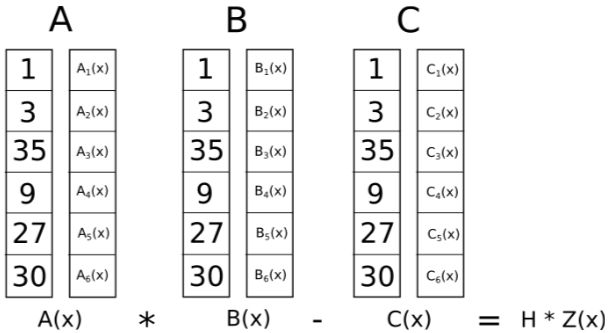# 剩余部分

### 关于椭圆曲线你需要知道以下知识：

1）椭圆曲线有一个 "生成点" G，G 做 n 次加法运算后可得到椭圆曲线上的所有点，n 称为椭圆曲线的阶。

2）当 d 足够大时，知道椭圆曲线上的两个点 P, Q 其中 Q = d*P，求 d 困难，这就是椭圆曲线上的离散对数问题。

1. e(aP, bQ) = e(P, Q)ab

2. e(P, Q+R) = e(P, Q)*e(P, R)

3. e(P+S, Q) = e(P, Q)*e(S, Q)

encrypt(p) = p*G = P，

P = p*G, Q = q*G, R = r*G

5*p + 7*q = 11 *r

5*P + 7*Q = 11 *R

e(P, Q)*e(G, G*5)=1

p*q + 5 = 0.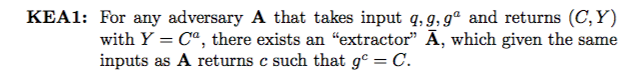### 下面我们看一下对椭圆曲线运算是怎样运用对运算的

e(R, Q) ?= e(P, S).

P1*i1+P2*i2+...+P10*i10，S 是点 Q1,Q2,...,Q10 使用同样系数的线性组合。我们可以使用同样的方法来验证 R*k = S.回顾一下我们刚刚讲的 QAP 的解是这种形式的：

A(x) * B(x) - C(x) = H(x) * Z(x).

πA = G * A(t), πA' = G*A(t)*ka

πB = G * B(t), πB' = G*B(t)*kb

πC = G * C(t), πC' = G*C(t)*kc

πH = G * H(t)

e(πA, πB)/e(πC, G)?=e(πH, G*Z(t))

# 关于讲师1.《Quadratic Arithmetic Programs: from Zero to Hero》

https://medium.com/@VitalikButerin/quadratic-arithmetic-programs-from-zero-to-hero-f6d558cea649

2.《Exploring Elliptic Curve Pairings 》

https://medium.com/@VitalikButerin/exploring-elliptic-curve-pairings-c73c1864e627

3.《Zk-SNARKs: Under the Hood 》

https://medium.com/@VitalikButerin/zk-snarks-under-the-hood-b33151a013f6

0
0 收藏

### 作者的其它热门文章0 评论
0 收藏
0